# Stokes flow

Mark Mendl

## Homework Statement

Let a spherical object move through a fluid in R3. For slow velocities, assume Stokes’ equations apply. Take the point of view that the object is stationary and the fluid streams by. The setup for the boundary value problem is as follows: given U = (U, 0, 0), U constant, find u and p such that Stokes’ equation holds in the region exterior to a sphere of radius R, u = 0 on the boundary of the sphere and u = U at infinity.
The solution to this problem (in spherical coordinates centered in the object) is called Stokes’ Flow: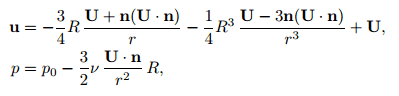where p0 is constant and n = r/r .
(a) Verify this solution.
(b) Show that the drag is 6πRνU and there is no lift.

If someone can help it would be great.

## The Attempt at a Solution

a) [/B]I started using the stokes equations but couldn't get there.

Mentor
a) I started using the stokes equations but couldn't get there.

What coordinate system did you use, and what form of the stokes equations did you use? How can we help if you don't show us what you have done so far?

Chet

Mark Mendl
What coordinate system did you use, and what form of the stokes equations did you use? How can we help if you don't show us what you have done so far?

Chet
Using spherical coordinates (that is what asked in the problem I guess) and these equations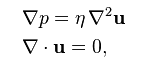Mark Mendl
I'm not sure about the n (U.n) term, it stays just U/r2?

Thanks,
Mark

Mentor
Using spherical coordinates (that is what asked in the problem I guess) and these equations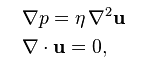OK. Express these equations in spherical coordinates, of course without the longitudinal dependence because of symmetry.

Chet

Mark Mendl
OK. Express these equations in spherical coordinates, of course without the longitudinal dependence because of symmetry.

Chet
To prove the divergence equal to zero, only the term in r exists, so we have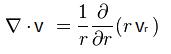I solved that but it's not 0, I guess the problem is in the n (U.n) term...

Mentor
To prove the divergence equal to zero, only the term in r exists, so we have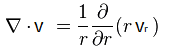I solved that but it's not 0, I guess the problem is in the n (U.n) term...
This is not correct for a couple of reasons. First of all, you have written the equation for the divergence in cylindrical coordinates, rather than spherical coordinates. Secondly, you have omitted the derivative with respect to the latitudinal coordinate.

Also, please write down for me the spherical components of the fluid velocity vector in the radial direction and latitudinal direction.

Chet

Mark Mendl
This is not correct for a couple of reasons. First of all, you have written the equation for the divergence in cylindrical coordinates, rather than spherical coordinates. Secondly, you have omitted the derivative with respect to the latitudinal coordinate.

Also, please write down for me the spherical components of the fluid velocity vector in the radial direction and latitudinal direction.

Chet
Yes, actually when I solved it I used the equation for spherical and not that one, I put it wrong. (it's with both r squared).
But I only used the radial direction in the fluid velocity... how do we have a latitudinal component if n and U only have terms in r?

Mark Mendl
.

Last edited:
Mentor
Yes, actually when I solved it I used the equation for spherical and not that one, I put it wrong. (it's with both r squared).
But I only used the radial direction in the fluid velocity... how do we have a latitudinal component if n and U only have terms in r?
The components of U they gave are for cartesian coordinates. I guess they should have mentioned that. You need to convert this to spherical coordinates.

Chet

Mark Mendl
The components of U they gave are for cartesian coordinates. I guess they should have mentioned that. You need to convert this to spherical coordinates.

Chet
So I rewrite U = (Ucosθ, Usinθ, 0), my vr will be something like -3/4*R*Ucosθ(1/r+1/r3) - 1/4R3*Ucosθ(1/r3-3/r5)+Ucosθ ?

Mentor
So I rewrite U = (Ucosθ, Usinθ, 0), my vr will be something like -3/4*R*Ucosθ(1/r+1/r3) - 1/4R3*Ucosθ(1/r3-3/r5)+Ucosθ ?
No. It looks like you have several algebra errors in there. Try again, or show more details please.

Chet

Mark Mendl
Hum... how would stay n(U.n)? cos(θ)U/r? probably not...

Mentor
Hum... how would stay n(U.n)? cos(θ)U/r? probably not...
No. n is the unit vector in the radial direction. So n(U.n)=Ucosθn.

Chet